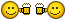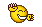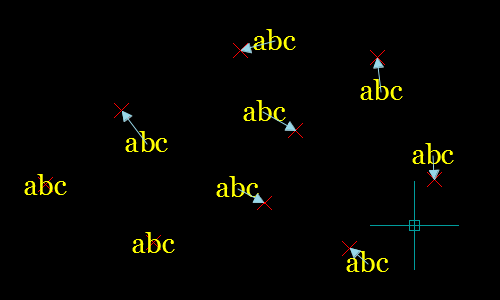# Moving Text Block to adjacent point## Recommended PostsI use a very useful LISP by Pedro Ferreira called PMSFptwtxt which places a 3D point at the insertion point of a text string. It's great for processing point surveys which have been flattened, thus losing their elevation height.

Unfortunately the text label giving z-values for each point is usually not positioned correctly relative to the point which it describes, so the 3D points created are correct in z-value but not x and y. Most labels are consistently located (say top right) at a fixed distance from the point, but a few are below left or above centre etc. Having gone through labouriously finding and moving any rougue labels by eye, I started thinking there might be a more efficient way to acheive this.

If there was a recursive LISP that draws a line between the text insertion point and it's neighbouring point then I could filter the line lengths to find the atypical distances and fix these manually, then select the remaining labels and move them all together. The only tricky bit would be telling the LISP which point the text relates to. I'm guessing this could be done with some sort of delaunay/voronoi routine to find the nearest neighbour. I'm a LISP noob, so I'm not even sure if this is possible but any hints/opinions would be gratefully received...

Tom

• Replies 26
• Created

#### Top Posters In This Topic

•8

•3

•3

•2

#### Top Posters In This Topic

•Lee Mac 8 posts

•HaroldA 3 posts

•urbat 3 posts

•Least 2 posts

#### Popular PostsWhy does the LISP have to be recursive?   Give this a try:   ;; Text 2 Point - Lee Mac 2012 ;; Prompts for a selection of Text and Point entities and moves ;; each Text entity to

#### Posted ImagesWhy does the LISP have to be recursive?

Give this a try:

```;; Text 2 Point  -  Lee Mac 2012
;; Prompts for a selection of Text and Point entities and moves
;; each Text entity to the nearest (2D distance) Point entity in the set.
;;
;; Retains existing Text elevation.

(defun c:txt2pt ( / _textinsertion di1 di2 dxf ent inc ins lst mpt pnt sel txt )

(defun _textinsertion ( elist )
(if
(and
(zerop (cdr (assoc 72 elist)))
(zerop (cdr (assoc 73 elist)))
)
(cdr (assoc 10 elist))
(cdr (assoc 11 elist))
)
)

(if (setq sel (ssget "_:L" '((0 . "POINT,TEXT"))))
(progn
(repeat (setq inc (sslength sel))
(setq ent (entget (ssname sel (setq inc (1- inc)))))
(if (eq "POINT" (cdr (assoc 0 ent)))
(setq lst (cons (cdr (assoc 10 ent)) lst))
(setq txt (cons (cons (_textinsertion ent) ent) txt))
)
)
(foreach ent txt
(setq ins (list (caar ent) (cadar ent)))
(if (setq pnt (vl-some '(lambda ( pnt ) (equal ins (list (car pnt) (cadr pnt)) 1e-8)) lst))
(setq lst (vl-remove pnt lst))
(progn
(setq di1 (distance ins (list (caar lst) (cadar lst)))
mpt (car lst)
)
(foreach pnt (cdr lst)
(if (< (setq di2 (distance ins (list (car pnt) (cadr pnt)))) di1)
(setq di1 di2
mpt pnt
)
)
)
dxf (cdr ent)
dxf (subst (cons 10 pnt) (assoc 10 dxf) dxf)
dxf (subst (cons 11 pnt) (assoc 11 dxf) dxf)
)
(entmod dxf)
(setq lst (vl-remove mpt lst))
)
)
)
)
)
(princ)
)
```

It will prompt for a selection of Text and Point entities and move each Text entity to the nearest Point entity (nearest by 2D distance), unless a Point is already found with equal X/Y coords as the Text entity.

The program will retain the existing elevation of the Text entity.

Example:

It's probably not the most efficient routine, but I don't have time study a better algorithm.

Edited by Lee Mac
•1
##### Share on other sitesAbsolutely perfect!

(and much quicker than my line drawing idea)

Thank you Lee Mac.

T

##### Share on other sitesYou're welcome##### Share on other sites
• 4 years later...Why does the LISP have to be recursive?

Give this a try:

```[color=GREEN];; Text 2 Point  -  Lee Mac 2012[/color]
[color=GREEN];; Prompts for a selection of Text and Point entities and moves[/color]
[color=GREEN];; each Text entity to the nearest (2D distance) Point entity in the set.[/color]
[color=GREEN];;[/color]
[color=GREEN];; Retains existing Text elevation.[/color]

([color=BLUE]defun[/color] c:txt2pt ( [color=BLUE]/[/color] _textinsertion di1 di2 dxf ent inc ins lst mpt pnt sel txt )

([color=BLUE]defun[/color] _textinsertion ( elist )
([color=BLUE]if[/color]
([color=BLUE]and[/color]
([color=BLUE]zerop[/color] ([color=BLUE]cdr[/color] ([color=BLUE]assoc[/color] 72 elist)))
([color=BLUE]zerop[/color] ([color=BLUE]cdr[/color] ([color=BLUE]assoc[/color] 73 elist)))
)
([color=BLUE]cdr[/color] ([color=BLUE]assoc[/color] 10 elist))
([color=BLUE]cdr[/color] ([color=BLUE]assoc[/color] 11 elist))
)
)

([color=BLUE]if[/color] ([color=BLUE]setq[/color] sel ([color=BLUE]ssget[/color] [color=MAROON]"_:L"[/color] '((0 . [color=MAROON]"POINT,TEXT"[/color]))))
([color=BLUE]progn[/color]
([color=BLUE]repeat[/color] ([color=BLUE]setq[/color] inc ([color=BLUE]sslength[/color] sel))
([color=BLUE]setq[/color] ent ([color=BLUE]entget[/color] ([color=BLUE]ssname[/color] sel ([color=BLUE]setq[/color] inc ([color=BLUE]1-[/color] inc)))))
([color=BLUE]if[/color] ([color=BLUE]eq[/color] [color=MAROON]"POINT"[/color] ([color=BLUE]cdr[/color] ([color=BLUE]assoc[/color] 0 ent)))
([color=BLUE]setq[/color] lst ([color=BLUE]cons[/color] ([color=BLUE]cdr[/color] ([color=BLUE]assoc[/color] 10 ent)) lst))
([color=BLUE]setq[/color] txt ([color=BLUE]cons[/color] ([color=BLUE]cons[/color] (_textinsertion ent) ent) txt))
)
)
([color=BLUE]foreach[/color] ent txt
([color=BLUE]setq[/color] ins ([color=BLUE]list[/color] ([color=BLUE]caar[/color] ent) ([color=BLUE]cadar[/color] ent)))
([color=BLUE]if[/color] ([color=BLUE]setq[/color] pnt ([color=BLUE]vl-some[/color] '([color=BLUE]lambda[/color] ( pnt ) ([color=BLUE]equal[/color] ins ([color=BLUE]list[/color] ([color=BLUE]car[/color] pnt) ([color=BLUE]cadr[/color] pnt)) 1e-) lst))
([color=BLUE]setq[/color] lst ([color=BLUE]vl-remove[/color] pnt lst))
([color=BLUE]progn[/color]
([color=BLUE]setq[/color] di1 ([color=BLUE]distance[/color] ins ([color=BLUE]list[/color] ([color=BLUE]caar[/color] lst) ([color=BLUE]cadar[/color] lst)))
mpt ([color=BLUE]car[/color] lst)
)
([color=BLUE]foreach[/color] pnt ([color=BLUE]cdr[/color] lst)
([color=BLUE]if[/color] ([color=BLUE]<[/color] ([color=BLUE]setq[/color] di2 ([color=BLUE]distance[/color] ins ([color=BLUE]list[/color] ([color=BLUE]car[/color] pnt) ([color=BLUE]cadr[/color] pnt)))) di1)
([color=BLUE]setq[/color] di1 di2
mpt pnt
)
)
)
dxf ([color=BLUE]cdr[/color] ent)
dxf ([color=BLUE]subst[/color] ([color=BLUE]cons[/color] 10 pnt) ([color=BLUE]assoc[/color] 10 dxf) dxf)
dxf ([color=BLUE]subst[/color] ([color=BLUE]cons[/color] 11 pnt) ([color=BLUE]assoc[/color] 11 dxf) dxf)
)
([color=BLUE]entmod[/color] dxf)
([color=BLUE]setq[/color] lst ([color=BLUE]vl-remove[/color] mpt lst))
)
)
)
)
)
([color=BLUE]princ[/color])
)
```

It will prompt for a selection of Text and Point entities and move each Text entity to the nearest Point entity (nearest by 2D distance), unless a Point is already found with equal X/Y coords as the Text entity.

The program will retain the existing elevation of the Text entity.

Example:

[ATTACH]33660[/ATTACH]

It's probably not the most efficient routine, but I don't have time study a better algorithm.

Hi Lee,

I am trying to achieve the same but I need to also move the text level to the point's level.

Do you have a lsp routine to do this by any chance?Thank you.

Harold

##### Share on other sitesHi Lee,

I am trying to achieve the same thing but I need to also move the text to the point's level.

Do you have a lsp routine that do this by any chance?Thank you.

Harold

##### Share on other sitesI am trying to achieve the same thing but I need to also move the text to the point's level.

Hi Harold,

Welcome to CADTutorIn the above code, simply change:

`(setq pnt (list (car mpt) (cadr mpt) (caddar ent))`

to:

`(setq pnt mpt`

This should achieve the desired result.

Lee

##### Share on other sitesThank you Lee.##### Share on other sitesNo worries!##### Share on other sites
• 1 month later...i fed searching for this over the internet and finally found this thread. my requirement is instead of moving to a point it should be move to nearest snap point like end or mid likewise. off topic question is there any options to create my own snap points like mid or end. Thank you.

##### Share on other sites
• 1 year later...On 3/15/2012 at 4:23 PM, Lee Mac said:

Why does the LISP have to be recursive?

Give this a try:

```
[color=GREEN];; Text 2 Point  -  Lee Mac 2012[/color]
[color=GREEN];; Prompts for a selection of Text and Point entities and moves[/color]
[color=GREEN];; each Text entity to the nearest (2D distance) Point entity in the set.[/color]
[color=GREEN];;[/color]
[color=GREEN];; Retains existing Text elevation.[/color]

([color=BLUE]defun[/color] c:txt2pt ( [color=BLUE]/[/color] _textinsertion di1 di2 dxf ent inc ins lst mpt pnt sel txt )

([color=BLUE]defun[/color] _textinsertion ( elist )
([color=BLUE]if[/color]
([color=BLUE]and[/color]
([color=BLUE]zerop[/color] ([color=BLUE]cdr[/color] ([color=BLUE]assoc[/color] 72 elist)))
([color=BLUE]zerop[/color] ([color=BLUE]cdr[/color] ([color=BLUE]assoc[/color] 73 elist)))
([color=BLUE]cdr[/color] ([color=BLUE]assoc[/color] 10 elist))
([color=BLUE]cdr[/color] ([color=BLUE]assoc[/color] 11 elist))
)
)

([color=BLUE]if[/color] ([color=BLUE]setq[/color] sel ([color=BLUE]ssget[/color] [color=MAROON]"_:L"[/color] '((0 . [color=MAROON]"POINT,TEXT"[/color]))))
([color=BLUE]progn[/color]
([color=BLUE]repeat[/color] ([color=BLUE]setq[/color] inc ([color=BLUE]sslength[/color] sel))
([color=BLUE]if[/color] ([color=BLUE]eq[/color] [color=MAROON]"POINT"[/color] ([color=BLUE]cdr[/color] ([color=BLUE]assoc[/color] 0 ent)))
([color=BLUE]setq[/color] lst ([color=BLUE]cons[/color] ([color=BLUE]cdr[/color] ([color=BLUE]assoc[/color] 10 ent)) lst))
([color=BLUE]setq[/color] txt ([color=BLUE]cons[/color] ([color=BLUE]cons[/color] (_textinsertion ent) ent) txt))
)
)
([color=BLUE]foreach[/color] ent txt
([color=BLUE]setq[/color] ins ([color=BLUE]list[/color] ([color=BLUE]caar[/color] ent) ([color=BLUE]cadar[/color] ent)))
([color=BLUE]if[/color] ([color=BLUE]setq[/color] pnt ([color=BLUE]vl-some[/color] '([color=BLUE]lambda[/color] ( pnt ) ([color=BLUE]equal[/color] ins ([color=BLUE]list[/color] ([color=BLUE]car[/color] pnt) ([color=BLUE]cadr[/color] pnt)) 1e-) lst))
([color=BLUE]setq[/color] di1 ([color=BLUE]distance[/color] ins ([color=BLUE]list[/color] ([color=BLUE]caar[/color] lst) ([color=BLUE]cadar[/color] lst)))
mpt ([color=BLUE]car[/color] lst)
)
([color=BLUE]if[/color] ([color=BLUE]<[/color] ([color=BLUE]setq[/color] di2 ([color=BLUE]distance[/color] ins ([color=BLUE]list[/color] ([color=BLUE]car[/color] pnt) ([color=BLUE]cadr[/color] pnt)))) di1)
([color=BLUE]setq[/color] di1 di2
mpt pnt
)
)
)
```

It will prompt for a selection of Text and Point entities and move each Text entity to the nearest Point entity (nearest by 2D distance), unless a Point is already found with equal X/Y coords as the Text entity.

The program will retain the existing elevation of the Text entity.

Example:

It's probably not the most efficient routine, but I don't have time study a better algorithm.

##### Share on other siteshello , i want to take that lisp to move points with diffrent distances
i copy that text and save it as .lsp and when i app load

##### Share on other sites14 hours ago, mohamed_ashraf said:

hello , i want to take that lisp to move points with diffrent distances
i copy that text and save it as .lsp and when i app load

I've updated the code in my post to remove the BBCode and correct the 1e-8 issues following the "upgrade" of the forum software.

##### Share on other sites
• 3 months later...Reference linie would be nice##### Share on other sites3 hours ago, urbat said:

Reference linie would be niceNot sure what you mean?##### Share on other sitesor similar##### Share on other sites11 hours ago, urbat said:

This actually only requires a relatively small modification -

```;; Text 2 Point  -  Lee Mac 2012
;; Prompts for a selection of Text and Point entities and moves
;; each Text entity to the nearest (2D distance) Point entity in the set.
;;
;; Retains existing Text elevation.

(defun c:txt2pt ( / _textinsertion di1 di2 dxf ent inc ins lst mpt pnt sel txt )

(defun _textinsertion ( elist )
(if
(and
(zerop (cdr (assoc 72 elist)))
(zerop (cdr (assoc 73 elist)))
)
(cdr (assoc 10 elist))
(cdr (assoc 11 elist))
)
)

(if (setq sel (ssget "_:L" '((0 . "POINT,TEXT"))))
(progn
(repeat (setq inc (sslength sel))
(setq ent (entget (ssname sel (setq inc (1- inc)))))
(if (eq "POINT" (cdr (assoc 0 ent)))
(setq lst (cons (cdr (assoc 10 ent)) lst))
(setq txt (cons (cons (_textinsertion ent) ent) txt))
)
)
(foreach ent txt
(setq ins (list (caar ent) (cadar ent)))
(if (setq pnt (vl-some '(lambda ( pnt ) (equal ins (list (car pnt) (cadr pnt)) 1e-8)) lst))
(setq lst (vl-remove pnt lst))
(progn
(setq di1 (distance ins (list (caar lst) (cadar lst)))
mpt (car lst)
)
(foreach pnt (cdr lst)
(if (< (setq di2 (distance ins (list (car pnt) (cadr pnt)))) di1)
(setq di1 di2
mpt pnt
)
)
)
;;;                             dxf (cdr ent)
;;;                             dxf (subst (cons 10 pnt) (assoc 10 dxf) dxf)
;;;                             dxf (subst (cons 11 pnt) (assoc 11 dxf) dxf)
)
;;;                       (entmod dxf)
(entmake (list '(0 . "LINE") (cons 10 ins) (cons 11 pnt)))
(setq lst (vl-remove mpt lst))
)
)
)
)
)
(princ)
)

##### Share on other sitesBut text don't move.

I want to see where the text moves.

THX

##### Share on other sites
• 4 months later...Good afternoon,

Can this LSP be modified to move a block reference in a similar manner? My problem is with surveyed points where the blocks, for manholes, trees, etc, always come in at zero elevation, however a node comes in at the correct elevation. I would like the blocks to move to the same elevation as the nodes. The insertion point of the block sits in the same horizontal position as the node.

Thanks,

Chris

##### Share on other sites2 hours ago, Chris Hall said:

Good afternoon,

Can this LSP be modified to move a block reference in a similar manner? My problem is with surveyed points where the blocks, for manholes, trees, etc, always come in at zero elevation, however a node comes in at the correct elevation. I would like the blocks to move to the same elevation as the nodes. The insertion point of the block sits in the same horizontal position as the node.

Thanks,

Chris

Try this adaption of Lee's code. Minimally tested.

```(vl-load-com)

(defun c:blk2pt ( / ss fuzz cnt obj p_lst b_lst blk pt i_pt dist d)

(setq ss (ssget ":L" '((0 . "POINT,INSERT")))
fuzz 1.0e-6
);end_setq

(cond (ss
(repeat (setq cnt (sslength ss))
(setq obj (vlax-ename->vla-object (ssname ss (setq cnt (1- cnt)))))
(if (wcmatch (strcase (vlax-get-property obj 'objectname)) "*POINT")
(setq p_lst (cons (vlax-get obj 'coordinates) p_lst))
(setq b_lst (cons (list (vlax-get obj 'insertionpoint) obj) b_lst))
);end_if
);end_repeat
(foreach pr b_lst
(setq blk (cadr pr) pt (car pr) i_pt nil)
(cond ( (setq i_pt (vl-some '(lambda (x) (equal pt x fuzz)) p_lst))
(setq p_lst (vl-remove i_pt p_lst))
(vlax-put blk 'insertionpoint i_pt)
)
(t  (setq i_pt (car p_lst)
dist (distance pt (reverse (cdr (reverse i_pt))))
);end_setq
(foreach p (cdr p_lst)
(if (< (setq d (distance pt (reverse (cdr (reverse p))))) dist)
(setq i_pt p dist d)
);end_if
);end_foreach
(setq p_lst (vl-remove i_pt p_lst))
(vlax-put blk 'insertionpoint i_pt)
)
);end_cond
);end_foreach
)
);end_cond
(princ)
);end_defun
```

## Join the conversation

You can post now and register later. If you have an account, sign in now to post with your account.
Note: Your post will require moderator approval before it will be visible.×   Pasted as rich text.   Restore formatting

Only 75 emoji are allowed.